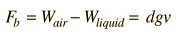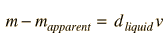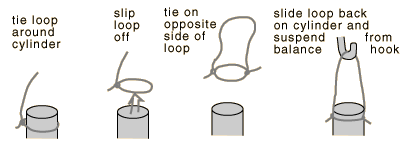# Density and Buoyancy

Objectives:

To learn the methods for measuring the density of

1. liquids and regularly shaped solids by direct measurement of mass and volume
2. solids by indirect volume measurement
3. liquids and irregularly shaped solids (e.g. mineral samples) by using Archimedes principle.

To become acquainted with measuring instruments and the estimation of measurement error.

Introduction

Mass is a physical property which all objects possess, but objects of the same size can have different masses and weights. This difference is characterized by another property, density. Density is defined as the ratio of an object's mass to its volume:

d= m/v
 Calculation
(1)

Since it is rare to find two different substances with identical densities, density is of value in helping to identify materials.

The specific gravity of a substance is defined as the ratio of the density of the substance to the density of water (1 gram/cm^3). This ratio is a convenient physical property since it has no units and is therefore independent of the system of measure you use to determine it.

Archimedes' principle states that the buoyant force experienced by a submerged object is equal to the weight of the liquid displaced by the object. Experimentally this appears in the fact that the submerged object apparently weighs less by an amount equal to the weight of the liquid displaced. The buoyant force can be expressed asCalculation
(2)

where d is the density of the liquid, g is the acceleration of gravity and v is the volume of the immersed object (or the immersed part of the body if it floats). In this experiment the pan balances will compare masses in grams rather than weights. Since W=mg, the apparent change in mass when submerged is(3)

Archimedes' principle will be used in two ways in this experiment:

1. 1. To determine the volume of an object by submerging it in a liquid of known density.
2. To determine the density of an unknown liquid by submerging an object of known volume and mass.
Procedure
 Data Sheet
 Equipment Needed

I. Determination of metal density by direct measurement of volume and mass.

1. Using the pan balance, determine and record the mass of the metal cylinder provided.
2. Use the vernier caliper to measure the length and diameter of the cylinder. Determine the volume in cm^3.
3. Calculate the density of the metal.

Archimedes' principle will be used in two ways in this experiment:

1. To determine the volume of an object by submerging it in a liquid of known density.
2. To determine the density of an unknown liquid by submerging an object of known volume and mass.

Procedure

I. Determination of metal density by direct measurement of volume and mass.

1. Using the pan balance, determine and record the mass of the metal cylinder provided.
2. Use the vernier caliper to measure the length and diameter of the cylinder. Determine the volume in cm^3.
3. Calculate the density of the metal.

Holding the string, lower the metal into the water until it is completely submerged. Record the new water level.

3. Determine the volume of the metal and recalculate the density using this volume and the mass from part I.

III. Testing of Archimedes' principle with metal sample.

1. Make use of the movable platform on the pan balance to support the graduated cylinder of water. Hang the metal cylinder on the hook above the pan so that the metal is suspended and submerged in the water without touching the sides. Determine its apparent mass using the scale. Find the difference between the actual and apparent masses and compare this to the mass of the water displaced using relationship (3).IV. Measurement of mineral sample densities using Archimedes principle.

1. Two mineral samples will be supplied. One is a light-colored mineral typical of the material of which the continents are made, and the other is a dark basaltic mineral characteristic of the ocean floors. Carefully determine the mass of each with the pan balance. This mass determination should be made while the rocks are dry- they will pick up a significant mass of water when wet.

2. Tie a light string on each sample so that they can be suspended from the hook above the pan of the balance. Fill a beaker with enough water to submerge the sample and use Archimedes principle to determine the density of each mineral.

V. Determination of liquid density by mass and volume measurement.

1. Determine the mass of your graduated cylinder while dry and then fill about half full with the unknown liquid supplied.

2. Measure the liquid volume and determine the liquid density.

VI. Determination of liquid density using Archimedes principle.

1. Suspend the cylindrical metal sample in the liquid as in part III and measure its apparent mass when submerged.

2. Use Archimedes principle to determine the liquid density.

## Data Sheet - Density and Buoyancy

I. Mass of metal ____________________

Length ____________________

Diameter ____________________

Volume ____________________ Density ____________________

II. Water level 1 ____________________

Water level 2 ____________________

Volume ____________________ Density ____________________

III. Apparent mass ____________________

Actual mass ____________________

Mass difference ___________ Mass of water displaced __________________

IV. Light Colored Mineral

Mass ____________________

Apparent mass ____________________

Volume ____________________ Density ____________________

Dark Mineral

Mass ____________________

Apparent mass ____________________

Volume ____________________ Density ____________________

Liquid mass ____________________

Liquid volume __________________ Density of liquid __________________

VI. Apparent mass of metal ____________________

Actual mass ____________________

Volume ____________________ Density of liquid ____________________

Questions:

1. Why does wood float? How can a steel barge float?

2. Equal volumes of lead and aluminum are submerged in water. Which feels the greatest buoyant force? Explain.

3. Would the Archimedes principle method give accurate densities for minerals with enclosed air bubbles? Explain.

## Equipment: Density and Buoyancy

 Short metal cylinder Vernier caliper Beaker String Light and dark rocks Unknown liquid Graduated cylinder Scales

Use envelopes to store metal cylinders with their names on them if the lab stretches over two days, so they won't have to re-measure the cylinder.

Index

Apparatus movie

Vernier caliper movie
HyperPhysics***** Physics 7110 Go Back